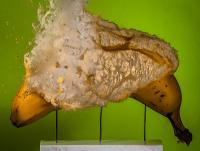# What would happen to a banana if all the radioactive potassium it contained were to decay simultaneously?

Mass of average banana: 118 grams

Potassium in average banana: 422 milligrams

• 0.0117% of which is radioactive potassium-40: 49.374 micrograms

Potassium-40 has an atomic mass of 6.64*10-23 grams: 7.43584337 * 1017 atoms of potassium-40

Each atom of potassium-40 can decay into either:

• Argon-40 10.72% of the time, releasing 1.5 mega electron volts
• Calcium-40 89.28% of the time, releasing 1.311 mega electron volts

So, 7.97122409 * 1016 atoms will decay into argon-40, releasing a total of 1.19568361 * 1017 mega electron volts (19156.96 joules)

And 6.63872096 * 1017 atoms will decay into calcium-40, releasing a total of 8.70336318 * 1017 mega electron volts (139443.24 joules)

Totaling to 158.6 kilo joules

We will assume a banana is mostly water (actually 74%, close enough)

Specific heat of water: 4.186 joules per gram

Room temperature is 25 celsius

The specific heat calculation tells us how much an object will heat up when exposed to energy: T = Q/(c * M)

158.6 kilo joules / (4.186 joules per gram * 118 grams) + 25 celsius = 346 celsius

If all the potassium-40 in a single banana were to decay simultaneously, it would reach over 300 degrees celsius and explode with the force of 38 grams of TNT.